# Flowchart and algorithm

24. May 2018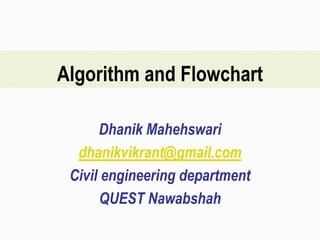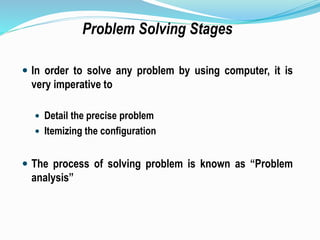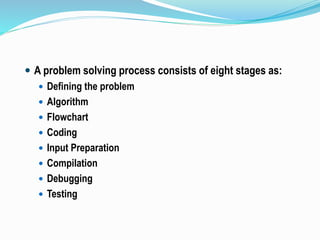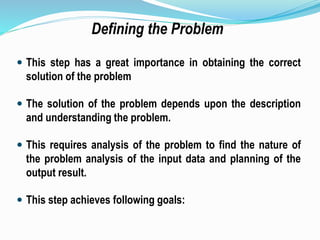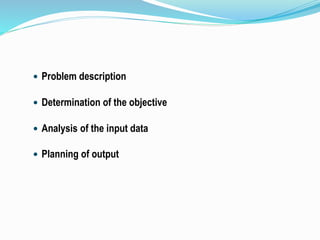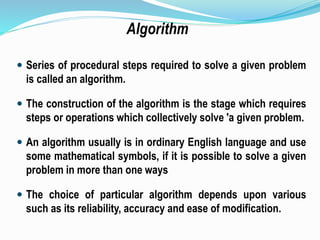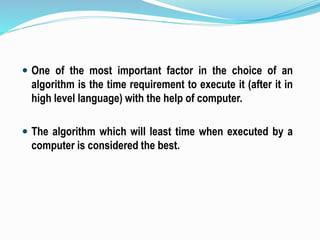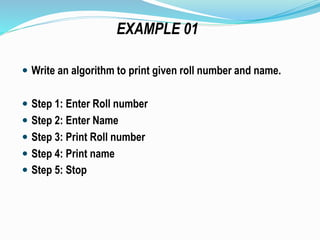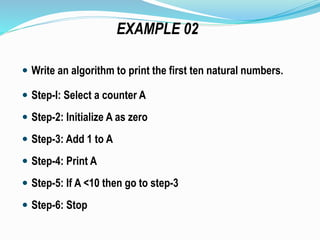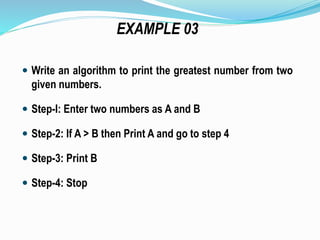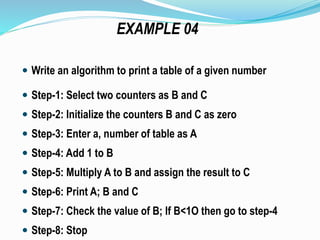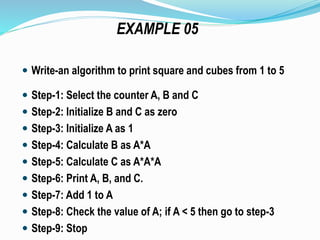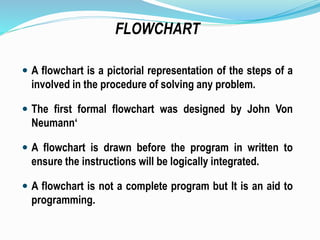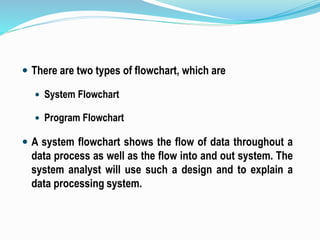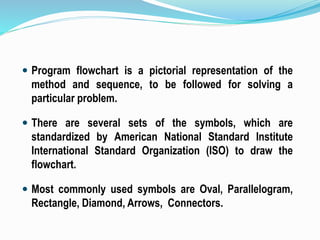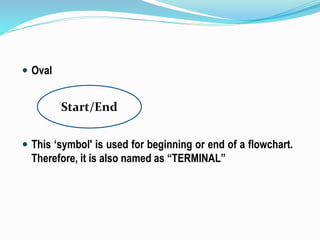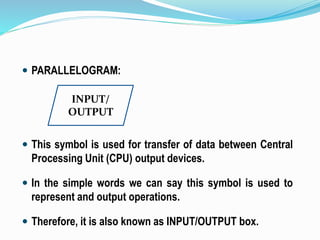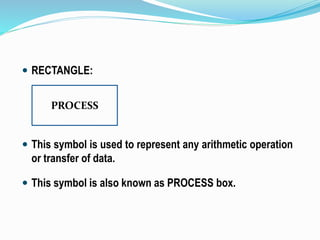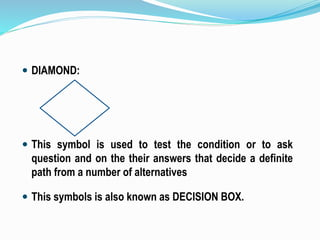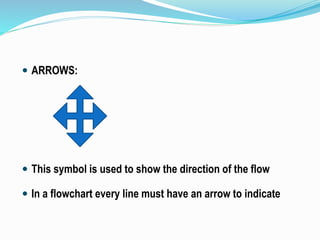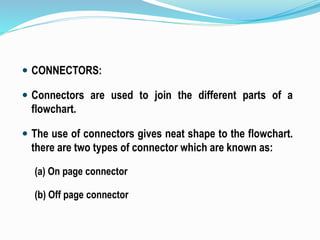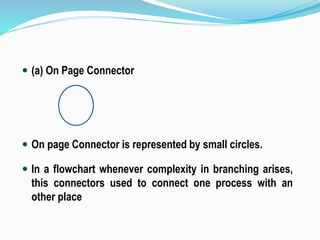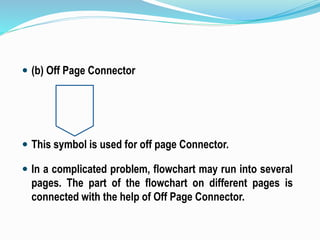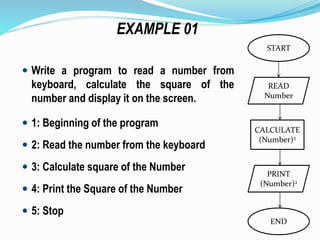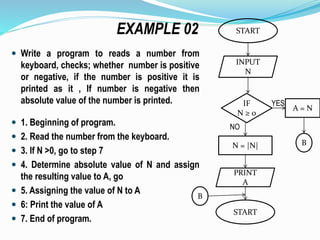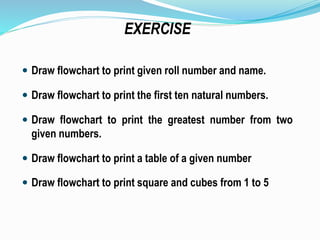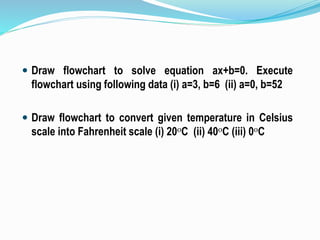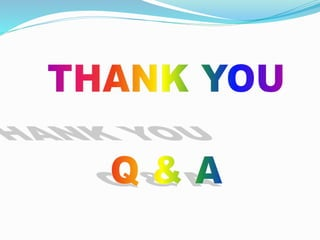1 von 28

### Flowchart and algorithm

• 1. Algorithm and Flowchart Dhanik Mahehswari dhanikvikrant@gmail.com Civil engineering department QUEST Nawabshah
• 2.  In order to solve any problem by using computer, it is very imperative to  Detail the precise problem  Itemizing the configuration  The process of solving problem is known as “Problem analysis” Problem Solving Stages
• 3.  A problem solving process consists of eight stages as:  Defining the problem  Algorithm  Flowchart  Coding  Input Preparation  Compilation  Debugging  Testing
• 4.  This step has a great importance in obtaining the correct solution of the problem  The solution of the problem depends upon the description and understanding the problem.  This requires analysis of the problem to find the nature of the problem analysis of the input data and planning of the output result.  This step achieves following goals: Defining the Problem
• 5.  Problem description  Determination of the objective  Analysis of the input data  Planning of output
• 6.  Series of procedural steps required to solve a given problem is called an algorithm.  The construction of the algorithm is the stage which requires steps or operations which collectively solve 'a given problem.  An algorithm usually is in ordinary English language and use some mathematical symbols, if it is possible to solve a given problem in more than one ways  The choice of particular algorithm depends upon various such as its reliability, accuracy and ease of modification. Algorithm
• 7.  One of the most important factor in the choice of an algorithm is the time requirement to execute it (after it in high level language) with the help of computer.  The algorithm which will least time when executed by a computer is considered the best.
• 8.  Write an algorithm to print given roll number and name.  Step 1: Enter Roll number  Step 2: Enter Name  Step 3: Print Roll number  Step 4: Print name  Step 5: Stop EXAMPLE 01
• 9.  Write an algorithm to print the first ten natural numbers.  Step-I: Select a counter A  Step-2: Initialize A as zero  Step-3: Add 1 to A  Step-4: Print A  Step-5: If A <10 then go to step-3  Step-6: Stop EXAMPLE 02
• 10.  Write an algorithm to print the greatest number from two given numbers.  Step-I: Enter two numbers as A and B  Step-2: If A > B then Print A and go to step 4  Step-3: Print B  Step-4: Stop EXAMPLE 03
• 11.  Write an algorithm to print a table of a given number  Step-1: Select two counters as B and C  Step-2: Initialize the counters B and C as zero  Step-3: Enter a, number of table as A  Step-4: Add 1 to B  Step-5: Multiply A to B and assign the result to C  Step-6: Print A; B and C  Step-7: Check the value of B; If B<1O then go to step-4  Step-8: Stop EXAMPLE 04
• 12.  Write-an algorithm to print square and cubes from 1 to 5  Step-1: Select the counter A, B and C  Step-2: Initialize B and C as zero  Step-3: Initialize A as 1  Step-4: Calculate B as A*A  Step-5: Calculate C as A*A*A  Step-6: Print A, B, and C.  Step-7: Add 1 to A  Step-8: Check the value of A; if A < 5 then go to step-3  Step-9: Stop EXAMPLE 05
• 13.  A flowchart is a pictorial representation of the steps of a involved in the procedure of solving any problem.  The first formal flowchart was designed by John Von Neumann‘  A flowchart is drawn before the program in written to ensure the instructions will be logically integrated.  A flowchart is not a complete program but It is an aid to programming. FLOWCHART
• 14.  There are two types of flowchart, which are  System Flowchart  Program Flowchart  A system flowchart shows the flow of data throughout a data process as well as the flow into and out system. The system analyst will use such a design and to explain a data processing system.
• 15.  Program flowchart is a pictorial representation of the method and sequence, to be followed for solving a particular problem.  There are several sets of the symbols, which are standardized by American National Standard Institute International Standard Organization (ISO) to draw the flowchart.  Most commonly used symbols are Oval, Parallelogram, Rectangle, Diamond, Arrows, Connectors.
• 16.  Oval  This ‘symbol' is used for beginning or end of a flowchart. Therefore, it is also named as “TERMINAL” Start/End
• 17.  PARALLELOGRAM:  This symbol is used for transfer of data between Central Processing Unit (CPU) output devices.  In the simple words we can say this symbol is used to represent and output operations.  Therefore, it is also known as INPUT/OUTPUT box. INPUT/ OUTPUT
• 18.  RECTANGLE:  This symbol is used to represent any arithmetic operation or transfer of data.  This symbol is also known as PROCESS box. PROCESS
• 19.  DIAMOND:  This symbol is used to test the condition or to ask question and on the their answers that decide a definite path from a number of alternatives  This symbols is also known as DECISION BOX.
• 20.  ARROWS:  This symbol is used to show the direction of the flow  In a flowchart every line must have an arrow to indicate
• 21.  CONNECTORS:  Connectors are used to join the different parts of a flowchart.  The use of connectors gives neat shape to the flowchart. there are two types of connector which are known as: (a) On page connector (b) Off page connector
• 22.  (a) On Page Connector  On page Connector is represented by small circles.  In a flowchart whenever complexity in branching arises, this connectors used to connect one process with an other place
• 23.  (b) Off Page Connector  This symbol is used for off page Connector.  In a complicated problem, flowchart may run into several pages. The part of the flowchart on different pages is connected with the help of Off Page Connector.
• 24.  Write a program to read a number from keyboard, calculate the square of the number and display it on the screen.  1: Beginning of the program  2: Read the number from the keyboard  3: Calculate square of the Number  4: Print the Square of the Number  5: Stop END START READ Number CALCULATE (Number)2 PRINT (Number)2 EXAMPLE 01
• 25.  Write a program to reads a number from keyboard, checks; whether number is positive or negative, if the number is positive it is printed as it , If number is negative then absolute value of the number is printed.  1. Beginning of program.  2. Read the number from the keyboard.  3. If N >0, go to step 7  4. Determine absolute value of N and assign the resulting value to A, go  5. Assigning the value of N to A  6: Print the value of A  7. End of program. EXAMPLE 02 START INPUT N PRINT A N = |N| NO IF N ≥ 0 A = N YES B START B
• 26.  Draw flowchart to print given roll number and name.  Draw flowchart to print the first ten natural numbers.  Draw flowchart to print the greatest number from two given numbers.  Draw flowchart to print a table of a given number  Draw flowchart to print square and cubes from 1 to 5 EXERCISE
• 27.  Draw flowchart to solve equation ax+b=0. Execute flowchart using following data (i) a=3, b=6 (ii) a=0, b=52  Draw flowchart to convert given temperature in Celsius scale into Fahrenheit scale (i) 20oC (ii) 40oC (iii) 0oC